# RD Sharma Solutions - Chapter 3 - Squares and Square Roots (Ex-3.1) Part - 2, Class 8 Math Notes | Study RD Sharma Solutions for Class 8 Mathematics - Class 8

## Class 8: RD Sharma Solutions - Chapter 3 - Squares and Square Roots (Ex-3.1) Part - 2, Class 8 Math Notes | Study RD Sharma Solutions for Class 8 Mathematics - Class 8

The document RD Sharma Solutions - Chapter 3 - Squares and Square Roots (Ex-3.1) Part - 2, Class 8 Math Notes | Study RD Sharma Solutions for Class 8 Mathematics - Class 8 is a part of the Class 8 Course RD Sharma Solutions for Class 8 Mathematics.
All you need of Class 8 at this link: Class 8

QUESTION 7: BY WHAT NUMBER SHOULD EACH OF THE FOLLOWING NUMBERS BE MULTIPLIED TO GET A PERFECT SQUARE IN EACH CASE? ALSO, FIND THE NUMBER WHOSE SQUARE IS THE NEW NUMBER.
(I) 8820
(II) 3675
(III) 605
(IV) 2880
(V) 4056
(VI) 3468
(VII) 7776

(i) 8820 = 2 x 2 x 3 x 3 x 5 x 7 x 7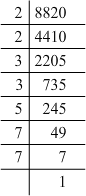Grouping them into pairs of equal factors:
8820 = (2 x 2) x (3 x 3) x (7 x 7) x 5
The factor, 5 is not paired. For a number to be a perfect square, each prime factor has to be paired. Hence, 8820 must be multiplied by 5 for it to be a perfect square.
The new number would be (2 x 2) x (3 x 3) x (7 x 7) x (5 x 5).
Furthermore, we have:
(2 x 2) x (3 x 3) x (7 x 7) x (5 x 5) = (2 x 3 x 5 x 7) x (2 x 3 x 5 x 7)
Hence, the number whose square is the new number is:
2 x 3 x 5 x 7 = 210
(ii) 3675 = 3 x 5 x 5 x 7 x 7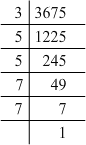Grouping them into pairs of equal factors:
3675 = (5 x 5) x (7 x 7) x 3
The factor, 3 is not paired. For a number to be a perfect square, each prime factor has to be paired. Hence, 3675 must be multiplied by 3 for it to be a perfect square.
The new number would be (5 x 5) x (7 x 7) x (3 x 3).
Furthermore, we have:
(5 x 5) x (7 x 7) x (3 x 3) = (3 x 5 x 7) x (3 x 5 x 7)
Hence, the number whose square is the new number is:
3 x 5 x 7 = 105
(iii) 605 = 5 x 11 x 11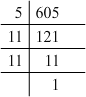Grouping them into pairs of equal factors:
605 = 5 x (11 x 11)
The factor, 5 is not paired. For a number to be a perfect square, each prime factor has to be paired. Hence, 605 must be multiplied by 5 for it to be a perfect square.
The new number would be (5 x 5) x (11 x 11).
Furthermore, we have:
(5 x 5) x (11 x 11) = (5 x 11) x (5 x 11)
Hence, the number whose square is the new number is:
5 x 11 = 55
(iv) 2880 = 2 x 2 x 2 x 2 x 2 x 2 x 3 x 3 x 5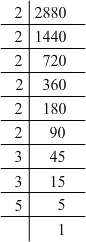Grouping them into pairs of equal factors:
2880 = (2 x 2) x (2 x 2) x (2 x 2) x (3 x 3) x 5
There is a 5 as the leftover. For a number to be a perfect square, each prime factor has to be paired. Hence, 2880 must be multiplied by 5 to be a perfect square.
The new number would be (2 x 2) x (2 x 2) x (2 x 2) x (3 x 3) x (5 x 5).
Furthermore, we have:
(2 x 2) x (2 x 2) x (2 x 2) x (3 x 3) x (5 x 5) = (2 x 2 x 2 x 3 x 5) x (2 x 2 x 2 x 3 x 5)
Hence, the number whose square is the new number is:
2 x 2 x 2 x 3 x 5 = 120
(v) 4056 = 2 x 2 x 2 x 3 x 13 x 13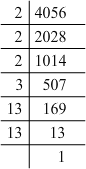Grouping them into pairs of equal factors:
4056 = (2 x 2) x (13 x 13) x 2 x 3
The factors at the end, 2 and 3 are not paired. For a number to be a perfect square, each prime factor has to be paired. Hence, 4056 must be multiplied by 6 (2 x 3) for it to be a perfect square.
The new number would be (2 x 2) x (2 x 2) x (3 x 3) x (13 x 13).
Furthermore, we have:
(2 x 2) x (2 x 2) x (3 x 3) x (13 x 13) = (2 x 2 x 3 x 13) x (2 x 2 x 3 x 13)
Hence, the number whose square is the new number is:
2 x 2 x 3 x 13 = 156
(vi) 3468 = 2 x 2 x 3 x 17 x 17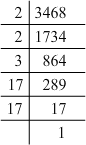Grouping them into pairs of equal factors:
3468 = (2 x 2) x (17 x 17) x 3
The factor 3 is not paired. For a number to be a perfect square, each prime factor has to be paired. Hence, 3468 must be multiplied by 3 for it to be a perfect square.
The new number would be (2 x 2) x (17 x 17) x (3 x 3).
Furthermore, we have:
(2 x 2) x (17 x 17) x (3 x 3) = (2 x 3 x 17) x (2 x 3 x 17)
Hence, the number whose square is the new number is:
2 x 3 x 17 = 102
(vii) 7776 = 2 x 2 x 2 x 2 x 2 x 3 x 3 x 3 x 3 x 3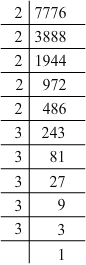Grouping them into pairs of equal factors:
7776 = (2 x 2) x (2 x 2) x (3 x 3) x (3 x 3) x 2 x 3
The factors, 2 and 3 at the end are not paired. For a number to be a perfect square, each prime factor has to be paired. Hence, 7776 must be multiplied by 6 (2 x 3) for it to be a perfect square.
The new number would be (2 x 2) x (2 x 2) x (2 x 2) x (3 x 3) x (3 x 3) x (3 x 3).
Furthermore, we have:
(2 x 2) x (2 x 2) x (2 x 2) x (3 x 3) x (3 x 3) x (3 x 3) = (2 x 2 x 2 x 3 x 3 x 3) x (2 x 2 x 2 x 3 x 3 x 3)
Hence, the number whose square is the new number is:
2 x 2 x 2 x 3 x 3 x 3 = 216

Question 8: By what numbers should each of the following be divided to get a perfect square in each case? Also, find the number whose square is the new number.
(i) 16562
(ii) 3698
(iii) 5103
(iv) 3174
(v) 1575

(i) 16562 = 2 x 7 x 7 x 13 x 13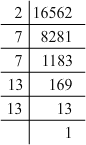Grouping them into pairs of equal factors:
16562 = 2 x (7 x 7) x (13 x 13)
The factor, 2 is not paired. For a number to be a perfect square, each prime factor has to be paired. Hence, 16562 must be divided by 2 for it to be a perfect square.
The new number would be (7 x 7) x (13 x 13).
Furthermore, we have:
(7 x 7) x (13 x 13) = (7 x 13) x (7 x 13)
Hence, the number whose square is the new number is:
7 x 13 = 91
(ii) 3698 = 2 x 43 x 43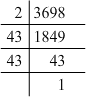Grouping them into pairs of equal factors:
3698 = 2 x (43 x 43)
The factor, 2 is not paired. For a number to be a perfect square, each prime factor has to be paired. Hence, 3698 must be divided by 2 for it to be a perfect square.
The new number would be (43 x 43).
Hence, the number whose square is the new number is 43.
(iii) 5103 = 3 x 3 x 3 x 3 x 3 x 3 x 7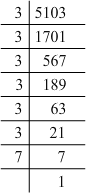Grouping them into pairs of equal factors:
5103 = (3 x 3) x (3 x 3) x (3 x 3) x 7
The factor, 7 is not paired. For a number to be a perfect square, each prime factor has to be paired. Hence, 5103 must be divided by 7 for it to be a perfect square.
The new number would be (3 x 3) x (3 x 3) x (3 x 3).
Furthermore, we have:
(3 x 3) x (3 x 3) x (3 x 3) = (3 x 3 x 3) x (3 x 3 x 3)
Hence, the number whose square is the new number is:
3 x 3 x 3 = 27
(iv) 3174 = 2 x 3 x 23 x 23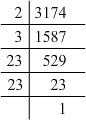Grouping them into pairs of equal factors:
3174 = 2 x 3 x (23 x 23)
The factors, 2 and 3 are not paired. For a number to be a perfect square, each prime factor has to be paired. Hence, 3174 must be divided by 6 (2 x 3) for it to be a perfect square.
The new number would be (23 x 23).

Hence, the number whose square is the new number is 23.
(v) 1575 = 3 x 3 x 5 x 5 x 7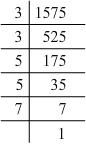Grouping them into pairs of equal factors:
1575 = (3 x 3) x (5 x 5) x 7
The factor, 7 is not paired. For a number to be a perfect square, each prime factor has to be paired. Hence, 1575 must be divided by 7 for it to be a perfect square.
The new number would be (3 x 3) x (5 x 5).
Furthermore, we have:
(3 x 3) x (5 x 5) = (3 x 5) x (3 x 5)
Hence, the number whose square is the new number is:
3 x 5 = 15

QUESTION 9: FIND THE GREATEST NUMBER OF TWO DIGITS WHICH IS A PERFECT SQUARE.
ANSWER 9: WE KNOW THAT 102 IS EQUAL TO 100 AND 92 IS EQUAL TO 81.
SINCE 10 AND 9 ARE CONSECUTIVE NUMBERS, THERE IS NO PERFECT SQUARE BETWEEN 100 AND 81. SINCE 100 IS THE FIRST PERFECT SQUARE THAT HAS MORE THAN TWO DIGITS, 81 IS THE GREATEST TWO-DIGIT PERFECT SQUARE.

Question 10: Find the least number of three digits which is perfect square.
Let us make a list of the squares starting from 1.
12 = 1
22 = 4
32 = 9
42 = 16
52 = 25
62 = 36
72 = 49
82 = 64
92 = 81
102 = 100
The square of 10 has three digits. Hence, the least three-digit perfect square is 100.

QUESTION 11: FIND THE SMALLEST NUMBER BY WHICH 4851 MUST BE MULTIPLIED SO THAT THE PRODUCT BECOMES A PERFECT SUQARE.
ANSWER 11: Prime factorisation of 4851:
4851 = 3 x 3 x 7 x 7 x 11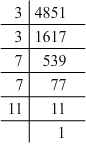Grouping them into pairs of equal factors:
4851 = (3 x 3) x (7 x 7) x 11
The factor, 11 is not paired. The smallest number by which 4851 must be multiplied such that the resulting number is a perfect square is 11.

QUESTION 12: FIND THE SMALLEST NUMBER BY WHICH 28812 MUST BE DIVIDED SO THAT THE QUOTIENT BECOMES A PERFECT SQUARE.
ANSWER 12: Prime factorisation of 28812:
28812 = 2 x 2 x 3 x 7 x 7 x 7 x 7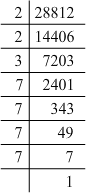Grouping them into pairs of equal factors:
28812 = (2 x 2) x (7 x 7) x (7 x 7) x 3
The factor, 3 is not paired. Hence, the smallest number by which 28812 must be divided such that the resulting number is a perfect square is 3.

QUESTION 13: FIND THE SMALLEST NUMBER BY WHICH 1152 MUST BE DIVIDED SO THAT IT BECOMES A PERFECT SQUARE. ALSO, FIND THE NUMBER WHOSE SQUARE IS THE RESULTING NUMBER.
Prime factorisation of 1152:
1152 = 2 x 2 x 2 x 2 x 2 x 2 x 2 x 3 x 3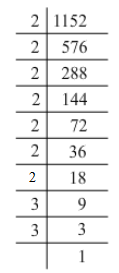Grouping them into pairs of equal factors:
1152 = (2 x 2) x (2 x 2) x (2 x 2) x (3 x 3) x 2
The factor, 2 at the end is not paired. For a number to be a perfect square, each prime factor has to be paired. Hence, 1152 must be divided by 2 for it to be a perfect square.

The resulting number would be (2 x 2) x (2 x 2) x (2 x 2) x (3 x 3).
Furthermore, we have:

(2 x 2) x (2 x 2) x (2 x 2) x (2 x 2) x (3 x 3) = (2 x 2 x 2 x 3) x (2 x 2 x 2 x 3)
Hence, the number whose square is the resulting number is:
2 x 2 x 2 x 3 = 24.

The document RD Sharma Solutions - Chapter 3 - Squares and Square Roots (Ex-3.1) Part - 2, Class 8 Math Notes | Study RD Sharma Solutions for Class 8 Mathematics - Class 8 is a part of the Class 8 Course RD Sharma Solutions for Class 8 Mathematics.
All you need of Class 8 at this link: Class 8Use Code STAYHOME200 and get INR 200 additional OFF

## RD Sharma Solutions for Class 8 Mathematics

88 docs

Track your progress, build streaks, highlight & save important lessons and more!

,

,

,

,

,

,

,

,

,

,

,

,

,

,

,

,

,

,

,

,

,

,

,

,

;